# Extended Energy Vector Prediction of Source Localisation Including Aspects of the Precedence Effect

Peter Stitt, Stéphanie Bertet and Maarten van Walstijn

contact: pstitt01 [at] qub.ac.uk

This is an accompanying webpage to ongoing research on energy vector prediction of localisation and providing supporting information to various publications.

If you use the work or code presented here, please cite reference  and this website.

## Introduction

The extended energy vector is defined by Stitt, Bertet and van Walstijn  aswhere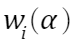is a weight that includes time-, level- and direction-dependent factors as a perceptual weight. It allows for the prediction of phantom source directions for listening conditions which include time differences between the loudspeaker signals arriving at the listener. The parameter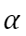determines how much of transient or stationary response is included and depends on stimulus type. For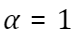it collapses to the original form of the energy vector from Gerzon . Low valuesshould be used for transient signals.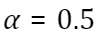has been found to give good results for ongoing noise stimuli. How this affects localisation with lower-order Ambisonics is demonstrated at the bottom of this page with localisation maps; the dependence on target angle can be appreciated with animated localisation maps.

This page gives examples of uses for the extended energy vector via Matlab scripts. The main Matlab code is available here.

When time differences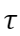are introduced the classic version of the energy vector does not predict any movement in the phantom source position.

Let's set the loudspeakers up in the kind of arrangement used in classic lead-lag precedence effect experiments:

clear

% make the lines thicker for the plots

set(0,'defaultlinelinewidth',3)

% loudspeaker gains are equal in lead-lag experiments

G = [1;1];

% loudspeaker delays - the right loudspeaker is delayed

tau = [0;2]*1e-3;

% loudspeaker angles

az = [45;-45]/180*pi;

% loudspeaker distances

dist = [2;2];

% loudspeaker coordinates

[ldspkCoords(:,1),ldspkCoords(:,2)] = pol2cart(az,dist);

And the extended and standard energy vectors are computed for this case, assuming a listener at the origin (sweet spot):

% listener coordinates

listCoords = [0,0];

% alpha parameter. Low values are used for transient stimulus, the kind normally used in lead-lag situations

alpha = 0.1;

% standard energy vector (obtained by setting alpha = 1)

E = enervecExt(G,ldspkCoords,listCoords,1,tau);

% extended energy vector

extE = enervecExt(G,ldspkCoords,listCoords,alpha,tau);

% plot the directions of the predicted perceived source direction for both vectors

figure

plot(ldspkCoords(:,1),ldspkCoords(:,2),'k.','MarkerSize',30);hold on

quiver(listCoords(1),listCoords(2),extE(1),extE(2),'LineWidth',2.5)

quiver(listCoords(1),listCoords(2),E(1),E(2),'r--','LineWidth',2.5)

ylabel('interaural axis (m)')

xlabel('displacement (m)')

legend('loudspeakers','extended E','standard E','location','SouthWest')

title(['\tau_{right} = ' num2str(tau(2)*1e3) ' ms, G_{right}/G_{left} = ' num2str(mag2db(G(2))) ' dB'])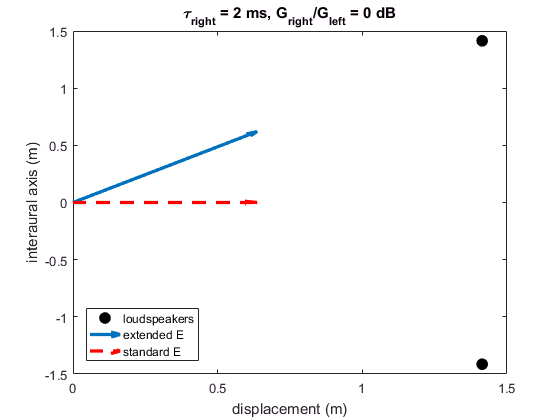This shows that the extended energy vector predicts better collapse of the phantom source into the leading loudspeaker. The standard energy vector shows no shift in the phantom source due to the addition of time delays.

For a range of time delay from 0 to 3 ms the predicted position for the extended energy vector is shown:

nTau = 50;

% delay of the right loudspeaker

tauR = linspace(0,3,nTau)*1e-3;

thetaE = zeros(nTau,1);

for iTau = 1:nTau

% loudspeaker delays the right loudspeaker is delayed

tau = [0;tauR(iTau)];

% extended energy vector

extE = enervecExt(G,ldspkCoords,listCoords,alpha,tau);

% the predicted angle

thetaE(iTau) = atan2(extE(2),extE(1))/pi*180;

end

figure

plot(tauR*1e3,thetaE,'LineWidth',3)

grid on

xlabel('lag delay (ms)')

ylabel('\theta_{E}')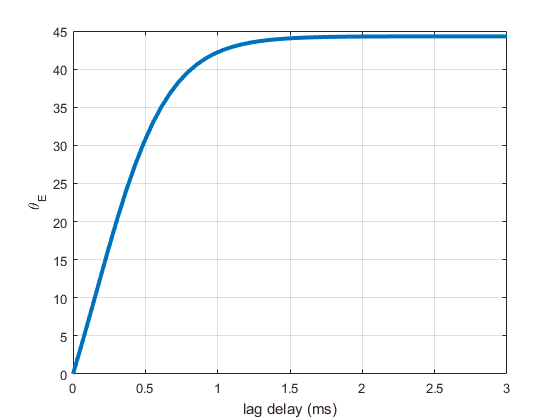The extended energy vector predicts the trends of the classic lead-lag experiments, with the phantom image moving almost fully to the leading loudspeaker direction over the first millisecond.

## Sitting Off-Centre with Stereo

More practically, the precedence effect is important for stereo when not sitting directly in the sweet spot. Here we look at a listener sitting 0.3 m left of the sweet spot

% listener coordinates

listCoords = [0,0.3];

% no imposed interchannel delay

tau = [0;0];

% standard energy vector

E = enervecExt(G,ldspkCoords,listCoords,1,tau);

% extended energy vector

extE = enervecExt(G,ldspkCoords,listCoords,alpha,tau);

figure

plot(ldspkCoords(:,1),ldspkCoords(:,2),'k.','MarkerSize',30);hold on

quiver(listCoords(1),listCoords(2),extE(1),extE(2),'LineWidth',2)

quiver(listCoords(1),listCoords(2),E(1),E(2),'r--','LineWidth',2)

ylabel('interaural axis (m)')

xlabel('displacement (m)')

legend('loudspeakers','extended E','standard E','location','SouthWest')

title(['\tau_{right} = ' num2str(tau(2)*1e3) ' ms, G_{right}/G_{left} = ' num2str(mag2db(G(2))) ' dB'])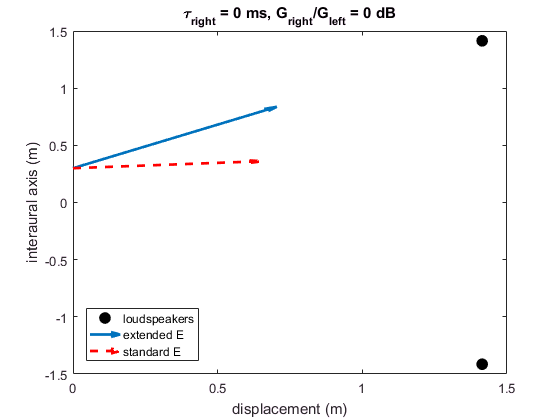This shows the well-known image shift to the nearest loudspeaker. For a very small shift from the sweet spot the image collapses fully into the leading loudspeaker. By contrast, the standard energy vector is only marginally shifted due to the gain changes from relative loudspeaker distances.

## Effect of Increasing Lag Gain

If the gain of the lagging loudspeaker is increased then the localisation dominance of the lead loudspeaker can be overcome. The effect of increasing loudspeaker gain is shown here:

nGain = 50;

% gain of the right loudspeaker

gainR = db2mag(linspace(0,18,nGain));

thetaE = zeros(nGain,1);

thetaEext = zeros(nGain,1);

% listener coordinates - listener in the sweet spot

listCoords = [0,0];

% impose a 2 ms delay on the right loudspeaker

tau = [0;2]*1e-3;

for iGain = 1:nGain

% loudspeaker gains

G = [1;gainR(iGain)];

% standard energy vector

E = enervecExt(G,ldspkCoords,listCoords,1,tau);

% energy vector

extE = enervecExt(G,ldspkCoords,listCoords,alpha,tau);

% the predicted angle of both vectors

thetaE(iGain) = atan2(E(2),E(1))/pi*180;

thetaEext(iGain) = atan2(extE(2),extE(1))/pi*180;

end

figure

plot(mag2db(gainR),thetaEext); hold on

plot(mag2db(gainR),thetaE)

grid on

xlabel('lag gain / lead gain (dB)')

ylabel('\theta_{E}')

legend('extended energy vector','standard energy vector')

title(['\tau_{right} = ' num2str(tau(2)*1e3) ' ms'])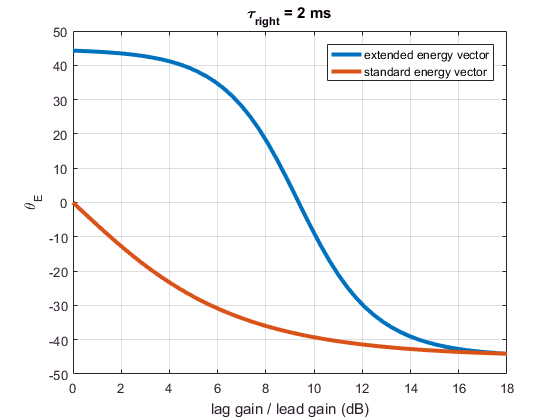For small increases in the lag gain the image direction is dominated by the lead before quickly moving to the lag direction. By 12 dB of increase the image has moved almost fully to the direction the lag direction. The standard energy vector again predicts no shift to the lead direction and moves to more intense loudspeaker direction as usual for a case without inter-channel time differences.

## Effect of Lag Direction

The extended energy vector includes a directional dependence so localisation dominance is weaker when the lag is near to the lead. If the lag starts near the lead and moves away it will pull the phantom source with it as it moves, before they are separated enought. After this point, localisation dominance eventually increases and the phantom source moves back to the lead direction. Here the lead loudspeaker is placed directly in front of the listener and the lag moved in a clockwise motion by 45 degrees.

% equal loudspeaker gains

G = [1;1];

nAz = 50;

% the azimuth of the lagging loudspeaker

% set the lead loudspeaker to 0 dg

[ldspkCoords(1,1),ldspkCoords(1,2)] = pol2cart(0,dist(1));

for iDir = 1:nAz

% the coordinates of the lag loudspeaker

[ldspkCoords(2,1),ldspkCoords(2,2)] = pol2cart(azLag(iDir),dist(2));

% standard energy vector

E = enervecExt(G,ldspkCoords,listCoords,1,tau);

% energy vector

extE = enervecExt(G,ldspkCoords,listCoords,alpha,tau);

% the predicted angle

thetaE(iDir) = atan2(E(2),E(1))/pi*180;

thetaEext(iDir) = atan2(extE(2),extE(1))/pi*180;

end

figure

plot(azLag/pi*180,thetaEext); hold on

plot(azLag/pi*180,thetaE)

grid on

xlabel('lag gain / lead gain (dB)')

ylabel('\theta_{E}')

legend('extended energy vector','standard energy vector','location','NorthWest')

title(['\tau_{right} = ' num2str(tau(2)*1e3) ' ms'])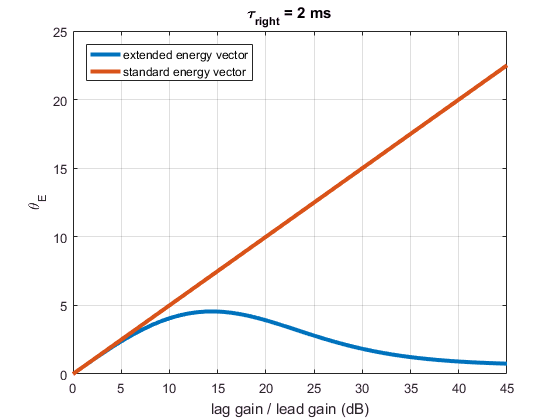The standard energy vector always predicts a perceived direction at the midpoint of the loudspeakers, while the extended energy vector shows the slight shift in direction before returning to the lead direction.

## Off-Centre Ambisonics

Ambisonics is a spatial audio system that recreates a sound field at the centre of the array. The size of the well-reproduced area is dependent on the order of the reconstruction, which defines the minimum number of loudspeakers required. The extended energy vector allows this to be evaluated from a perceptual perspective, instead of a physical reconstruction quality one.

In this example, an Ambisonic source is panned the full range 360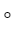around the array at the same distance as the loudspeakers. The stimulus is assumed to be non-transient noise, withbeing the most appropriate . The max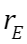weighting is used since it has been shown to give the best results of off-centre listeners.

clear ldspkCoords

% Ambisonic source angles

azAmbi = linspace(0,360,361)/180*pi;

% Ambisonic order

M = 1;

% number of loudspeakers in array

nLdspk = 2*M + 2;

% Array loudspeakers

azArray = linspace(0,360-360/nLdspk,nLdspk)/180*pi + pi/nLdspk ;

rArray = 2;

% Cartesian coordinates of array

[ldspkCoords(:,1),ldspkCoords(:,2)] = pol2cart(azArray,rArray);

% listener coordinates

listCoords = [-0.50,.5];

% alpha value to 0.5 for non-transient noise

alpha = 0.5;

% calculate the relative array directions

azRelArray = cart2pol(ldspkCoords(:,1)-listCoords(1),ldspkCoords(:,2)-listCoords(2));

for iAzAmbi = 1:361

% Ambisonic loudspeaker gains

G = ambiGains(azAmbi(iAzAmbi),M,azArray,1).';

% standard energy vector

E = enervecExt(G,ldspkCoords,listCoords,1);

% energy vector

extE = enervecExt(G,ldspkCoords,listCoords,alpha);

% the predicted angle

thetaE(iAzAmbi) = atan2(E(2),E(1));

thetaEext(iAzAmbi) = atan2(extE(2),extE(1));

% calculate the position of the intended source relative to the listener

[targCoords(1),targCoords(2)] = pol2cart(azAmbi(iAzAmbi),rArray);

thetaTarg(iAzAmbi) = cart2pol(targCoords(1)-listCoords(1),targCoords(2)-listCoords(2));

end

% unwrap the predictions and convert to degrees

thetaE = unwrap(thetaE)/pi*180;

thetaEext = unwrap(thetaEext)/pi*180;

thetaTarg = unwrap(thetaTarg)/pi*180;

azRelArray = mod(azRelArray,2*pi)/pi*180;

figure

plot(azAmbi/pi*180,thetaEext); hold on

plot(azAmbi/pi*180,thetaE)

plot(azAmbi/pi*180,thetaTarg)

plot([zeros(size(azRelArray)),360*ones(size(azRelArray))].',[azRelArray,azRelArray].','k--'); hold on

xlabel('Ambisonic panning angle (degrees)')

ylabel('predicted angle (degrees)')

axis([0 360 -45 405])

set(gca,'XTick',0:45:360,'YTick',-45:45:405)

grid on

legend('extended energy vector','standard energy vector','target','location','SouthEast')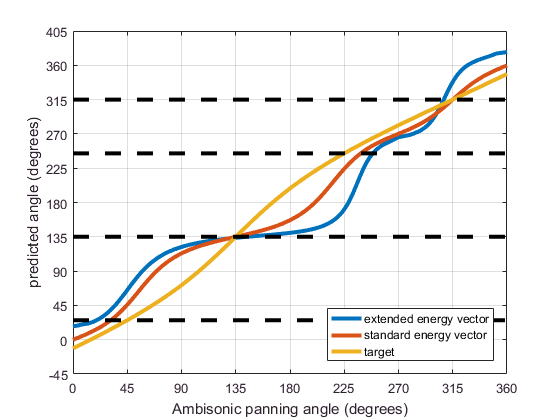The standard energy vector remains closer to the intended target source position, while the extended energy vector shows the image sticking close to the nearest loudspeakers (especially to the loudspeaker at 135).

## Localisation Maps

The extended energy vector has been shown to give more accurate results than the standard energy vector (and than two binaural models) . It can be used to plot the listening area within the loudspeaker array for either a particular set of loudspeaker gains, plus the corresponding error from the intended angle.

The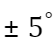contours are shown on the plot. The size of this region depends on the order of the system, shown for orders 1, 3 and 5. By order 5 the"sweet area" extends over most of the listening area.

% set up the evaluation grid

nX = 15; nY = nX;

x = linspace(-maxDisp,maxDisp,nX);

y = linspace(-maxDisp,maxDisp,nX);

[X,Y] = meshgrid(x,y);

% distance of the listening position from the origin

listenerDist = sqrt(X.^2 + Y.^2);

% run for order 1, 3 and 5

for M = [1,3,5]

clear ldspkCoords

% number of loudspeakers in array

nLdspk = 2*M + 2;

% Array loudspeakers

azArray = linspace(0,360-360/nLdspk,nLdspk)/180*pi;

rArray = 2;

% Cartesian coordinates of array

[ldspkCoords(:,1),ldspkCoords(:,2)] = pol2cart(azArray,rArray);

% image angle

imageAz = 30;

% target coordinates

targCoords = rArray*[cosd(imageAz), sind(imageAz)];

% Ambisonic loudspeaker gains

thetaEext = nan(size(X));

targRelAngle = nan(size(X));

extE = nan(nX,nY,2);

for iX = 1:nX % calculate the energy vector along x-direction

for iY = 1:nY % calculate the energy vector along y-direction

if listenerDist(iX,iY) > maxRadius

% if listener position is outside the defined maximum radius then don't calculate anything

else

% calculate the energy vector for each listening

% position

listCoords = [X(iX,iY),Y(iX,iY)];

extE(iX,iY,:) = enervecExt(G,ldspkCoords,listCoords,alpha);

thetaEext(iX,iY) = atan2(extE(iX,iY,2),extE(iX,iY,1));

% calculate the target direction relative to the listening

% positions

targetRelCoords = targCoords - [X(iX,iY),Y(iX,iY)];

[targRelAngle(iX,iY)] = cart2pol(targetRelCoords(1),targetRelCoords(2),0);

end

end

end

% calculate the error of the phantom image

% some plotting options

z0 = zeros(size(ldspkCoords(:,1)));

cdata = 200;

% the value (in degrees) to clip the error in the plot

clipError = 90;

% Plot the localisation map

figure

pcolor(x,y,Error); hold on

quiver(X,Y,squeeze(extE(:,:,1)),squeeze(extE(:,:,2)),'k')

colormap jet

hold on;

contour(x,y,Error,[-5,5],'k','LineWidth',1.5); hold on % [-180,-90,-60,-30,-15,-5,5,15,30,60,90,180]

p1 = patch(ldspkCoords(:,1),ldspkCoords(:,2),zeros(size(G)),cdata,'Marker','o','MarkerFaceColor','k','FaceColor','none','EdgeColor','none','MarkerSize',10);

p2 = patch(targCoords(1),targCoords(2),0,cdata,'Marker','o','MarkerFaceColor','r','FaceColor','none','EdgeColor','none','MarkerSize',8);

caxis([-clipError clipError])

ylabel('y (m) - left-right for listener')

xlabel('x (m) - front-back for listener')

title(['Order ' num2str(M) ' max rE - source position ' num2str(imageAz) '^{o}'])

set(gca,'FontSize',14,'XTick',-2:0.5:2,'YTick',-2:0.5:2)

axis equal

view(-90,90)

axis([-2.2 2.2 -2.2 2.2])

colormap jet

box on

grid on

cbar=colorbar;

ylabel(cbar,'Localisation error (degrees)')

end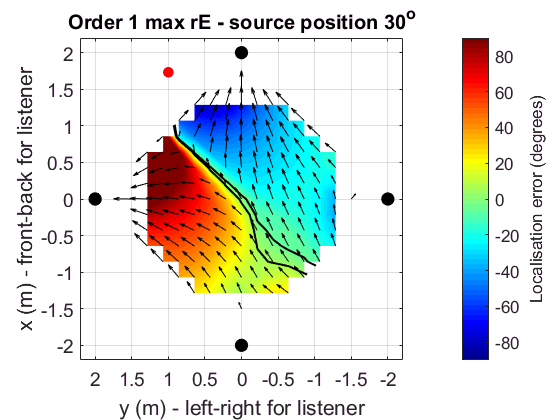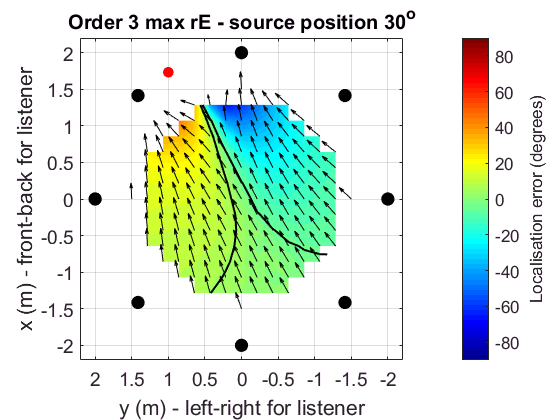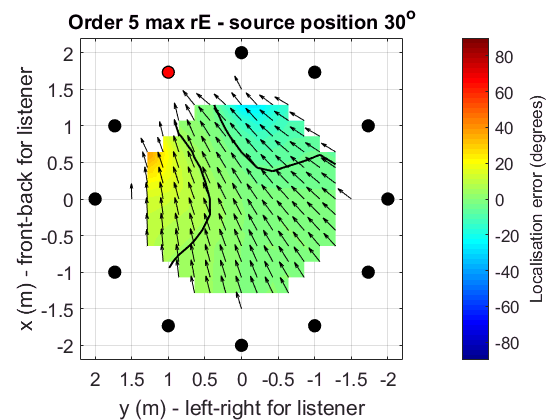## References

 P. Stitt, S. Bertet, and M. van Walstijn, “Extended energy vector prediction of ambisonically reproduced image direction at off-center listening positions,” J. Audio Eng. Soc., vol. 64, no. 5, pp. 299–310, 2016.

 M. Gerzon, “General metatheory of auditory localisation,” in 92nd Convention of the Audio Engineering Society, 1992, convention paper 3306.